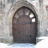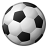# Calculate Field within Python script

326
4
11-07-2011 05:45 AMNew Contributor II
I need the help of the online community. I have a series of shapefile (polygons) representing forest cover and want to automate the following: add field, calculate field, select by attribute, select by location, calculate field. I'm using 9.3 and run my scripts with cygwin. I have been able to implement everything except the calculate fields. The script runs and kicks out after the calculate field (line 37) with no errors.

Any help is greatly appreciated as I'm not a programmer and I have tried suggestions from other posts and ESRI help docs.

```# ArcMap 9.3
# Import system modules
import sys, string, os, arcgisscripting

# Create the Geoprocessor object
gp = arcgisscripting.create()
gp.loghistory = False

#Set the input workspace where the files are to be processes are located
gp.Workspace = "C:\\Data\\ForConnect\\TEST"

try:
#List all feature classes and load the first one
fcs = gp.ListFeatureClasses()
fcs.reset()
fc = fcs.Next()

gp.overwriteoutput = 1

while fc:
Output_Layer = fc[:-4] + "_lyr"

print "Making feature layer: " + fc
# Process: Make Feature Layer...
gp.MakeFeatureLayer_management(fc, Output_Layer, "", "", "")

print "Adding fields: DISSOLVE and PROCESSED "
gp.addfield(Output_Layer, "DISSOLVE", "TEXT", "", "", "20", "", "", "", "")
gp.addfield(Output_Layer, "PROCESSED", "TEXT", "", "", "5", "", "", "", "")

print "Calculating field: " + Output_Layer + "\$PROCESSED "
# Process: Calculate Field
gp.CalculateField_management(Output_Layer, "PROCESSED", "\"NO\"", "PYTHON_9.3", "")

print "creating search cursor " + fc
rows = gp.searchcursor(fc, "", "", "", "")
row = rows.next() # get the first record
i = 1
while row:
rowid = row.ID

sba_exp = "\"ID\" = " + str(rowid) + " AND \"PROCESSED\" = \'NO\'"
dis_exp = str(int(row.ID)) + "_" + str(i)

print "Selecting records where " + sba_exp
# Process: Select Layer By Attribute...
gp.SelectLayerByAttribute_management(Output_Layer, "NEW_SELECTION", sba_exp) # selection type {NEW_SELECTION | ADD_TO_SELECTION | REMOVE_FROM_SELECTION | SUBSET_SELECTION | SWITCH_SELECTION | CLEAR_SELECTION}

print "Selecting records that touch the record of focus "

# Process: Calculate Field
print "Calculating field: DISSOLVE " + dis_exp
gp.CalculateField_management(Output_Layer, "DISSOLVE", dis_exp, "PYTHON_9.3", "")
print "Calculating fields: PROCESSED "
gp.CalculateField_management(Output_Layer, "PROCESSED", "\"YES\"", "PYTHON_9.3", "")

i = i + 1

row = rows.next()

fc = fcs.next()

except:
print gp.GetMessages (2)
print chr(7)
print "Exited with Errors.... Something is wrong",printime()

print 'Script has finished'```
Tags (3)
4 RepliesbyEsri Regular Contributor
Try this - use 'NO' within triple-quotes.

```gp.CalculateField_management(Output_Layer, "PROCESSED", """'NO'""", "PYTHON_9.3", "")
```New Contributor III
Matt :  did you tried changing
gp.CalculateField_management(Output_Layer, "DISSOLVE", dis_exp, "PYTHON_9.3", "")
"PYTHON_9.3" for "VB"?
gp.CalculateField_management(Output_Layer, "DISSOLVE", dis_exp, "VB", "")New Contributor II
Try this - use 'NO' within triple-quotes.

This didn't work; the script kicks out after the first calculate field. I also don't see how this would work as the python syntax shows the """'NO'""" as a comment.

Matt :  did you tried changing
gp.CalculateField_management(Output_Layer, "DISSOLVE", dis_exp, "PYTHON_9.3", "")
"PYTHON_9.3" for "VB"?
gp.CalculateField_management(Output_Layer, "DISSOLVE", dis_exp, "VB", "")

I have tried to use the "VB" option in another script I wrote with a series of if/else statements and since the rest of the script is written in python the gp didn't like the VB code. However I could have written the VB commands wrong.New Contributor II
Try this - use 'NO' within triple-quotes.

SOLUTION:
Based on Jose suggestion I changed the "PYTHON_9.3" to "VB" and left the new field value alone (eg, "\"NO\"") which uses python syntax. Thanks for the help.